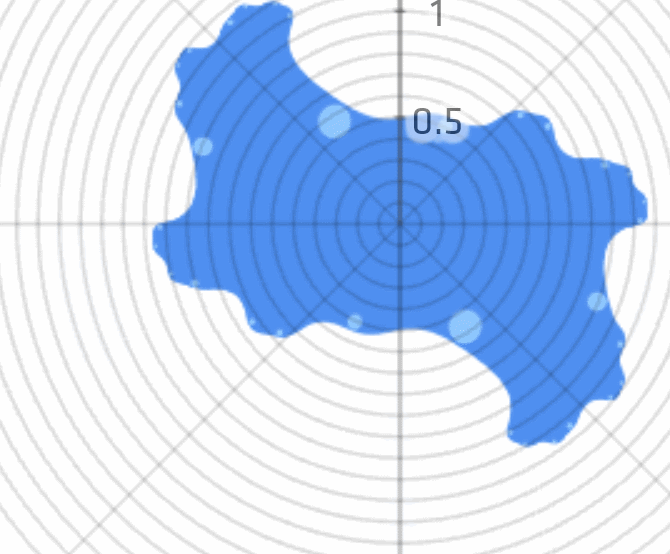# 3D Kaleidoscopic Fractals: Folding the Koch Snowflake

I came across a shader that generated a very pretty version of the Koch snowflake. I realized that after stepping through the code that the Koch snowflake is a good, simple example of generating fractals with folds. The code here is in the form of fragment shaders. For a shader primer, check out the Book of Shaders. The important thing is that to write a fragment shader, you build a function that takes a location (in our case, of a pixel) and returns a color. All the code here is “live”, though changes you make in each box will not cascade down to the subsequent ones.Generating the Koch curve this way is probably among the simplest examples of how fractals can be thought of as a repeated crumpling of space. The animation to the right is borrowed from a presentation by Steven Wittens that ably demonstrates this with a Julia fractal: squishing, translating and folding space progressively crumples up the boundary of the unit circle.

For the Koch curve, we need a different sort of fold. It’s easiest to understand the folding operation visually. I haven’t taken the time to justify this formula, but it’s a matter of vector arithmetic. You can adjust the angle to see how it creates a kaleidoscopic effect, reflecting the plane across a line through the origin.

Now we are going to start drawing a line. This formula calculates the distance to a line segment between $(0,0)$ and $(1,0)$. This is the line we’re going to fold up into a Koch curve.

There are two folds: first, across the angle $\frac{\pi}{6}$, and then horizontally.

We can iterate by moving and scaling the folded line to lie along the original line (from $(0,0)$ to $(0,1)$) and then folding the line up again.

And we can iterate this as many times as we’d like. It converges very rapidly, so you only need a handful of folds.

And just for fun, we can do two more folds to create two copies of the curve to create the Koch Snowflake.

A small adjustment and we get another familiar fractal, a Sierpinski triangle, or close enough. I recommend you adjust the number of iterations to get a feel for how it converges.

You might have worked this out already: I’ve been keeping something from you. The real motivation here is to justify a wider class of fractals, dubbed Kaleidoscopic Iterated Function Systems, or KIFS. KIFS are what you get when you start folding and scaling around different axes. A myriad of fractals fall out, and you can see why they’re called Kaleidoscopic- for the mirroring folds they’re built out of. Here’s an example. These reward fiddling: adjust the parameters to get a slightly better sense of how the folds affect the final output.

Another confession: In truth, we haven’t just been building a black and white image. We’ve been calculating a 2D distance estimator to the fractal. For any point in the plane, the value of our function is an approximation of the distance to the fractal. We can illustrate it by taking the modulo of the value:

Final confession: this distance estimator? It’s not useful. At least, not in 2D. But it’s exactly what we need to draw pictures of three-dimensional KIFS fractals. Raymarching is a rendering tool that can render pretty much any surface if you have an estimate of the distance to the surface from any point in space.

All we need to adjust is adding some folds that live inside the $yz$ plane, rather than keeping in the $xy$ plane. Taking that distance estimate and plugging it into a raymarcher, we get similar 3D fractals! Try adjusting the fold angles to get a sense of the sorts of shapes this is capable of.

You can play with the full source to this fractal here. This is a very simple and unoptimized renderer, and I’m not adept enough at writing raymarchers to tune it perfectly. More sophisticated and well-tuned renderers can draw much prettier pictures!

For more information you should check out my main source for this post, Mikael Hvidtfeldt Christensen’s series on distance estimated 3D fractals, which covers KIFS and more. His program Fragmentarium comes with a number of KIFS fractals built in, and produces some very pretty results.

< Previous: Building Escher's Square Limit in Pixels